Example Questions

Example Question #1 : How To Find The Solution To An Inequality With Subtraction

Find the slope of the inequality equation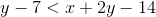–1

1

7

–7

0

–1

Explanation: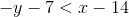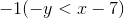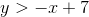From the equation we can see that the slope is –1.

Example Question #211 : Gre Quantitative Reasoningandare both integers.

If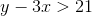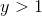, and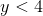, which of the following is a possible value of?Explanation:

Take the values of y that are possible, i.e. 2 and 3, and plug them into the first inequality. First, plug in 2. 2 – 3x > 21. Subtract 2 from both sides, and then divide by –3. Don't forget that when you divide or multiply by a negative number in an inequality you must flip the inequality sign. Thus, x < –19/3. Now plug in 3. We find, following the same steps, that when y=3, x < –6. Thus –7 is the correct answer.

Example Question #1 : How To Find The Solution To An Inequality With Subtraction

Quantity A:

The value(s) for which the following function is undefined: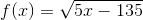Quantity B:Which of the following is true?

The two quantities are equal.

A comparison cannot be detemined from the given information.

Quantity B is larger.

Quantity A is larger.

Quantity B is larger.

Explanation:

This question is not as hard as it seems.  Remember that for real numbers, square roots cannot be taken of negative numbers.  Therefore, we know that this function is undefined for: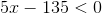This is simple to solve.  Merely add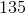to both sides: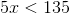Then, divide by: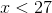Therefore, quantity A is less than quantity B.  This means that quantity B is greater than it.

Example Question #211 : Algebra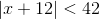Quantity A:Quantity B:Which of the following is true?

A comparison cannot be detemined from the given information.

The two quantities are equal.

Quantity B is larger.

Quantity A is larger.

A comparison cannot be detemined from the given information.

Explanation:

Recall that when you have an absolute value and an inequality like,

this is the same as saying that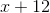must be between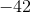and.  You can rewrite it: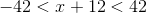To solve this, you merely need to subtractfrom all three values: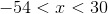Sinceis between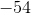and, it could be both larger or smaller than.  Therefore, you cannot determine the relationship based on the given information.

Example Question #1 : How To Find The Solution To An Inequality With Multiplication

Quantitative Comparison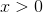Column A: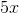Column B: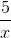The relationship cannot be determined from the information provided.

The quantities are equal.

Quantity A is greater.

Quantity B is greater.

The relationship cannot be determined from the information provided.

Explanation:

For quantitative comparison questions involving a shared variable between quantities, the best approach is to test a positive integer, a negative integer, and a fraction. Half of our work is eliminated, however, because the question stipulates that x > 0.  We only need to check a positive integer and a positive fraction between 0 and 1. Plugging in 2, we see that quantity A is greater than quantity B. Checking 1/2, however, we find that quantity B is greater than quantity A. Thus the relationship cannot be determined.

Example Question #1 : How To Find The Solution To An Inequality With Multiplication

If –1 < n < 1, all of the following could be true EXCEPT:

|n2 - 1| > 1

(n-1)2 > n

n2 < 2n

16n2 - 1 = 0

n2 < n

|n2 - 1| > 1

Explanation: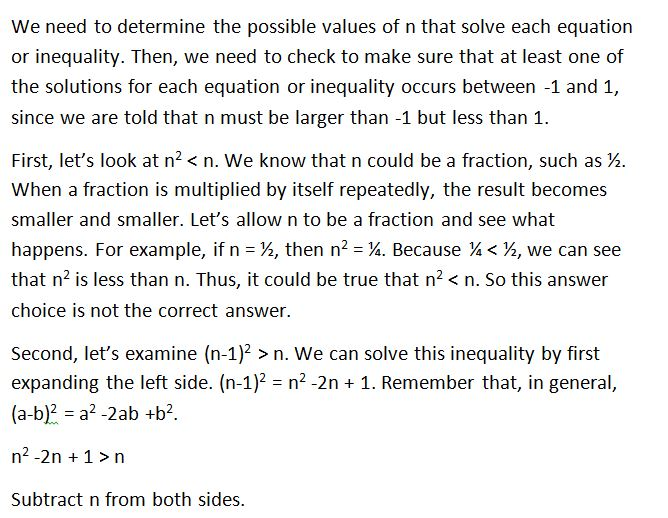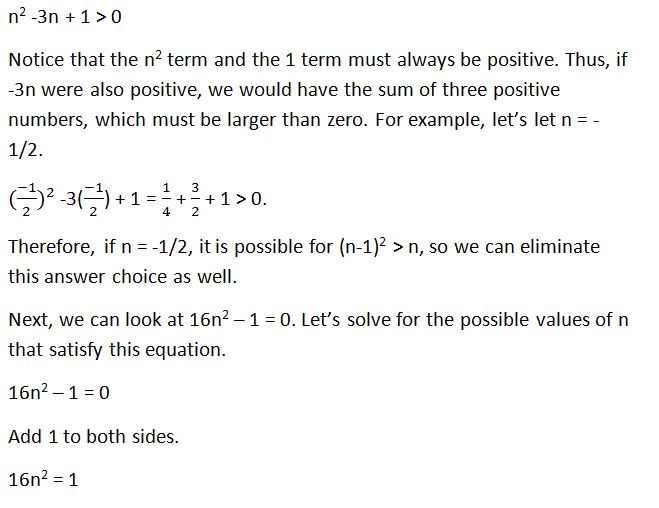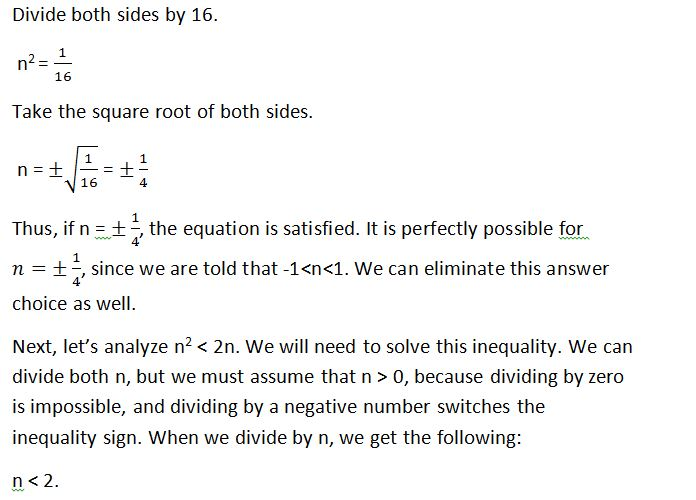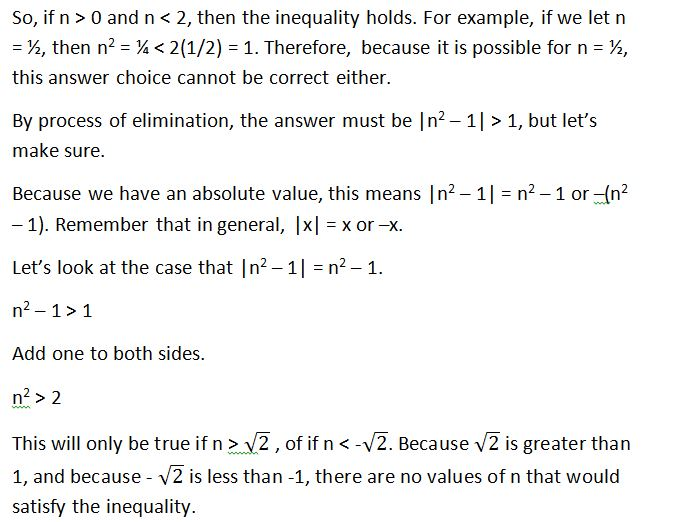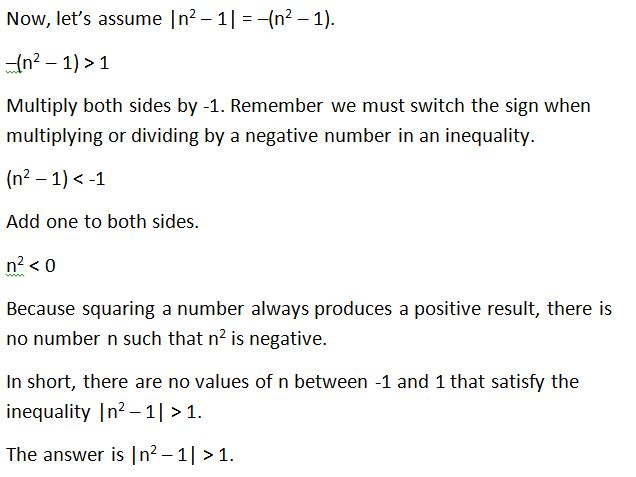Example Question #32 : Inequalities

(√(8) / -x ) <  2. Which of the following values could be x?

All of the answers choices are valid.

-3

-1

-2

-4

-1

Explanation:

The equation simplifies to x > -1.41. -1 is the answer.

Example Question #1 : How To Find The Solution To An Inequality With Multiplication

Solve for x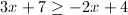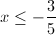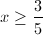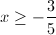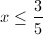Explanation: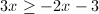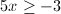Example Question #34 : Inequalities

We have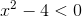, find the solution set for this inequality.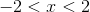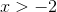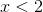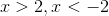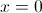Explanation: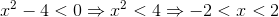Example Question #11 : Inequalities

Fill in the circle with either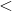,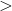, orsymbols: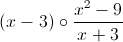for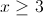.

The rational expression is undefined.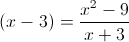None of the other answers are correct.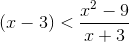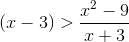Explanation:Let us simplify the second expression. We know that: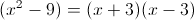So we can cancel out as follows: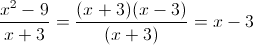Tired of practice problems?

Try live online GRE prep today.